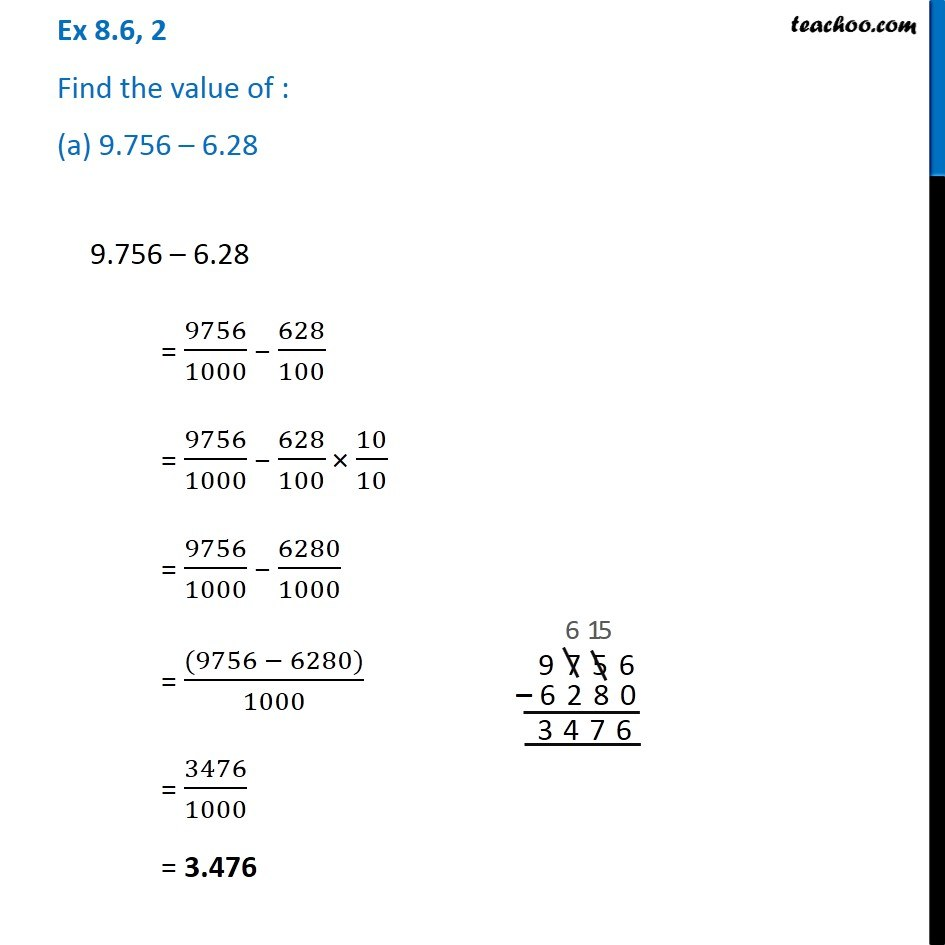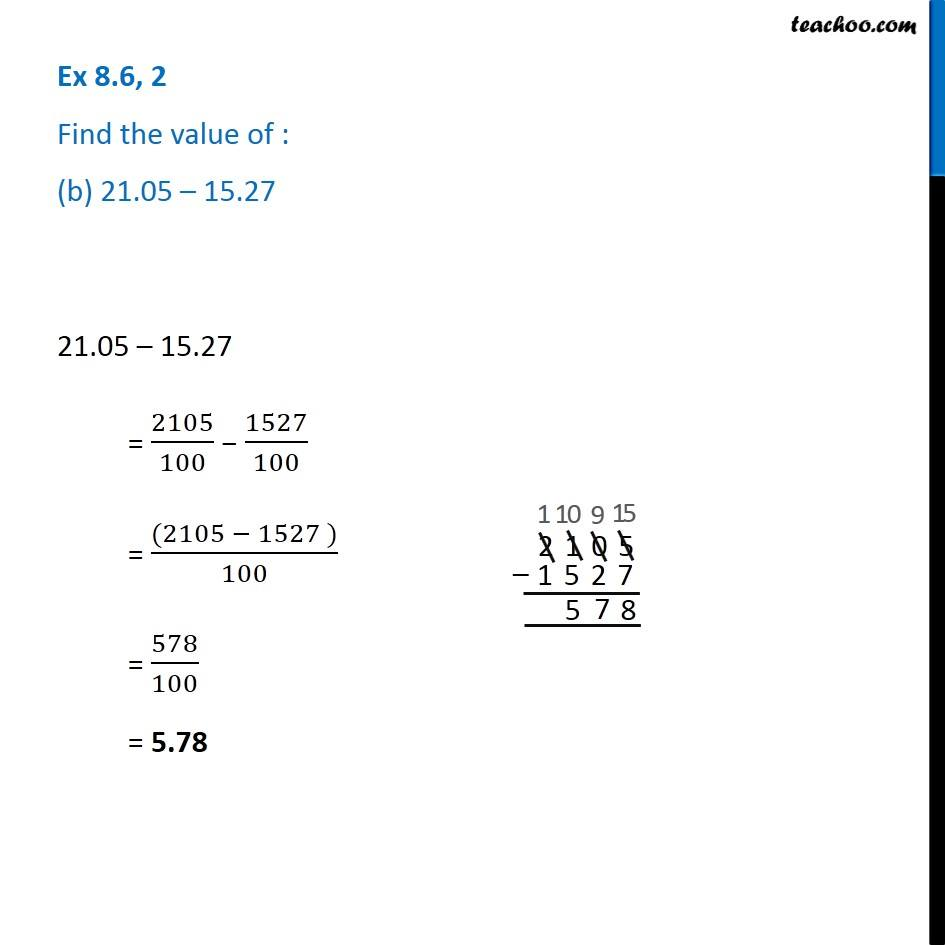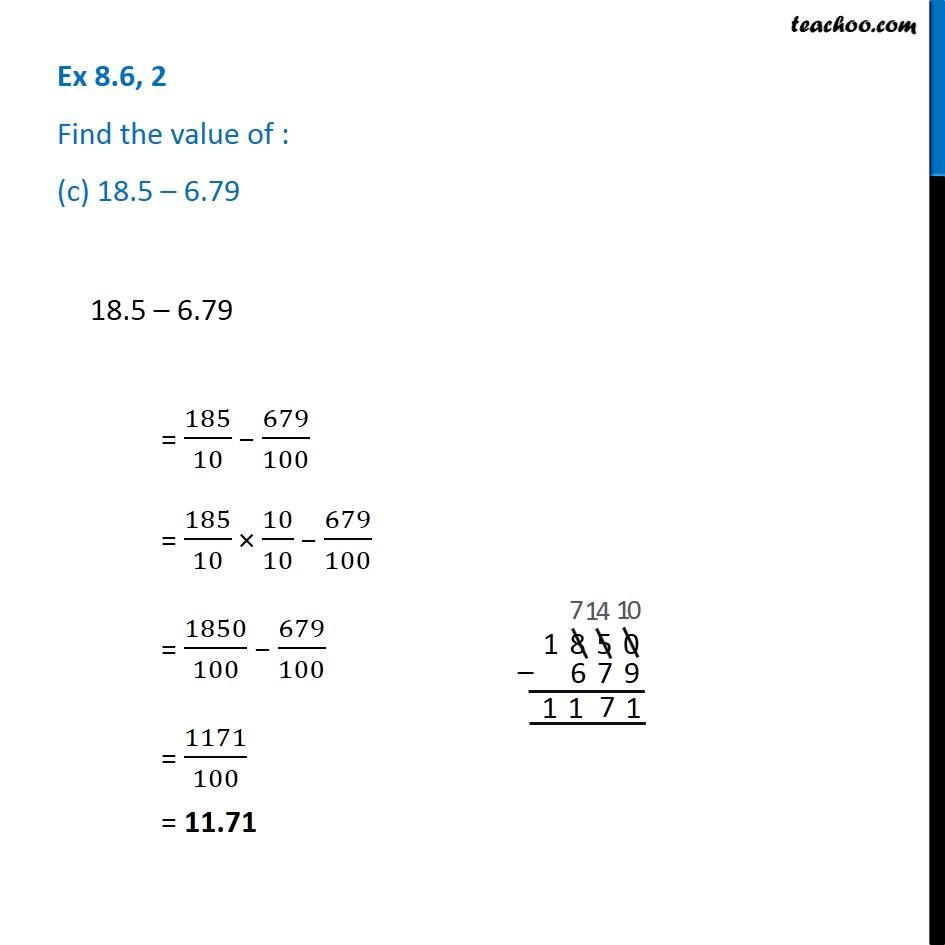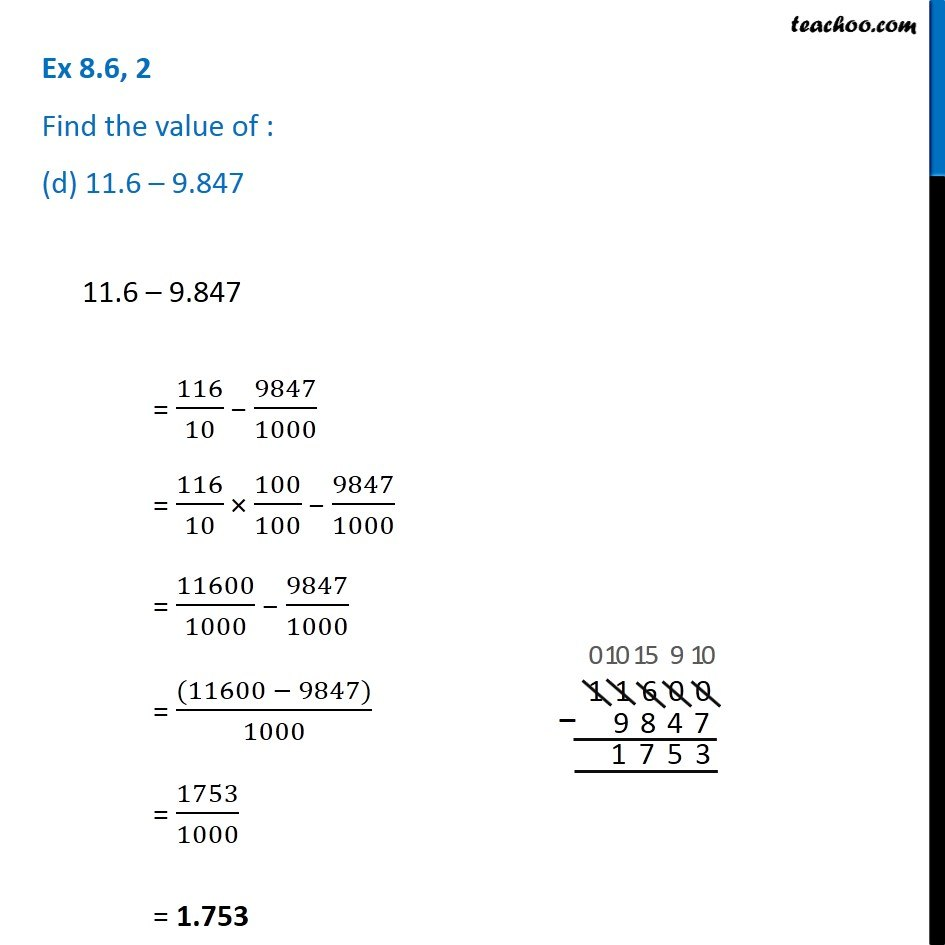Subscribe to our Youtube Channel - https://you.tube/teachoo

1. Chapter 8 Class 6 Decimals
2. Serial order wise
3. Ex 8.6

Transcript

Ex 8.6, 2 Find the value of : (a) 9.756 – 6.289.756 – 6.28 = 9756/1000 − 628/100 = 9756/1000 − 628/100 × 10/10 = 9756/1000 − 6280/1000 = ((9756 − 6280))/1000 = 3476/1000 = 3.476 Ex 8.6, 2 Find the value of : (b) 21.05 – 15.27 21.05 – 15.27 = 2105/100 − 1527/100 = ((2105 − 1527 ))/100 = 578/100 = 5.78 Ex 8.6, 2 Find the value of : (c) 18.5 – 6.7918.5 – 6.79 = 185/10 − 679/100 = 185/10 × 10/10 − 679/100 = 1850/100 − 679/100 = 1171/100 = 11.71 Ex 8.6, 2 Find the value of : (d) 11.6 – 9.84711.6 – 9.847 = 116/10 − 9847/1000 = 116/10 × 100/100 − 9847/1000 = 11600/1000 − 9847/1000 = ((11600 − 9847))/1000 = 1753/1000 = 1.753

Ex 8.6

Chapter 8 Class 6 Decimals
Serial order wise